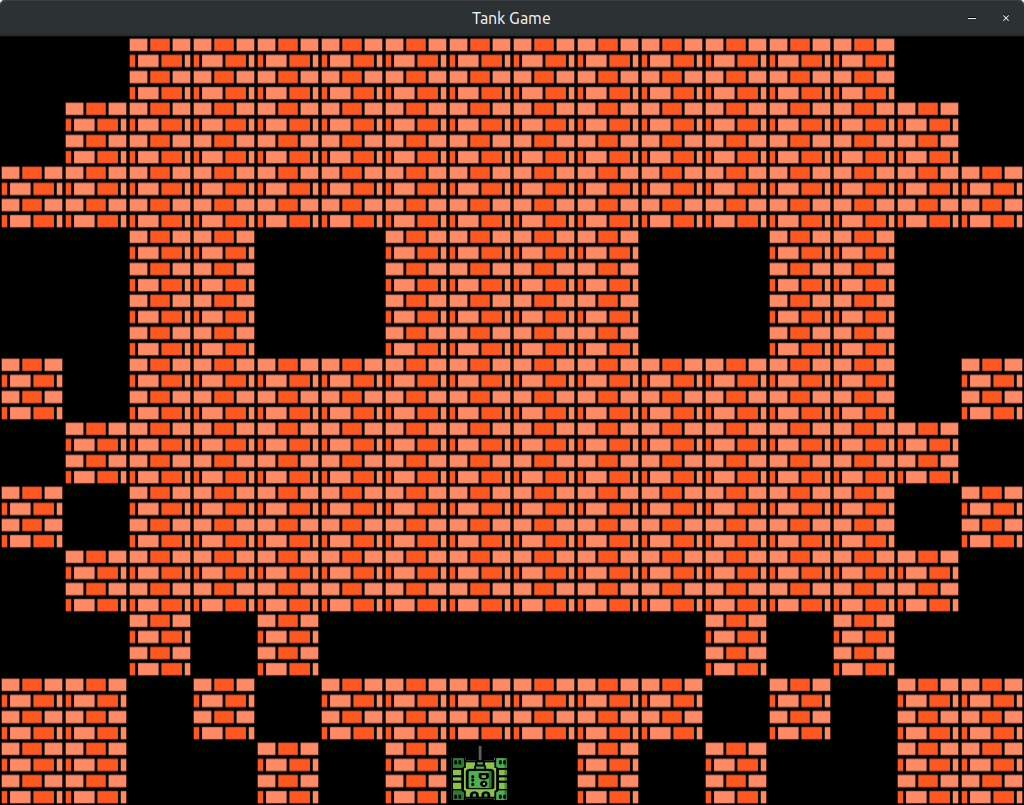#0

## 不同判斷的IF，相同的結果，如何減少重複程式碼？``````//外層if
if ( condition A 甲 )
{
//內層if
if ( condition B 甲 )
{
do the right thing~~~;
}
}
if ( condition A 乙 )
{
if ( condition B 乙 )
{
do the right thing~~~;
}
}
if ( condition A 丙 )
{
if ( condition B 丙 )
{
do the right thing~~~;
}
}
``````

``````switch (condition)
{
case A:
case B:
case C:
do the right thing~~~;
break;
}
``````

``````//外層if
if (condition A 甲 || condition A 乙 || condition A 丙)
{
//內層if
if (condition B 甲 || condition B 乙 || condition B 丙)
{
do the right thing~~~;
}
}
``````

## 謝謝用心回答的各位，最後我覺得List的解法算是與目的較為相似，其實這個問題本來就有點吹毛求疵，我主要是糾結在有沒有某種「邏輯」可以解決這樣的問題，但我想不出來，所以才上來問，再次感謝。

``````//外層if
if ((hitNum = iHitBlock(Vector2f(tank.left, tank.top)))!=-1)
{
//內層if
if (bInsideBlock(Vector2f(tank.left, tank.top),
sparrBrick[hitNum].getGlobalBounds()))
{
spTank.setPosition(origin);
return;
}
}
if ((hitNum = iHitBlock(Vector2f(tank.left+tank.width,tank.top)))!=-1){
if (bInsideBlock(Vector2f(tank.left+tank.width, tank.top),
sparrBrick[hitNum].getGlobalBounds()))
{
spTank.setPosition(origin);
return;
}
}
if ((hitNum = iHitBlock(Vector2f(tank.left+tank.width,
tank.top+tank.height)))!=-1)
{
if (bInsideBlock(Vector2f(tank.left+tank.width,
tank.top+tank.height),
sparrBrick[hitNum].getGlobalBounds()))
{
spTank.setPosition(origin);
return;
}
}
``````

（之所以這樣做是因為 坦克 和 磚塊 一樣大，如果坦克想從2個磚塊中間通過，很容易卡住，所以設計它就算超出一點點，壓到方塊也沒關係。）

### 4 個回答

1

``````Vector2f leftTop =  Vector2f(tank.left, tank.top)
Vector2f top = Vector2f(tank.left+tank.width,tank.top)
// ...其他
``````

``````if(iHitBlock(leftTop)!=-1){
// ...
}
if(iHitBlock(top)!=-1){
//...
}
//...
``````

``````if((hitNum = iHitBlock(Vector2f(tank.left, tank.top)))!=-1)
``````

`hitNum` 感覺可以不用賦值的吧？

``````if(iHitBlock(leftTop)!=-1)
``````

（就是這個function 不是 pure function ）

``````if (bInsideBlock(leftTop),
sparrBrick[iHitBlock(leftTop)].getGlobalBounds()))
``````

``````if(iHitBlock(leftTop)!=-1){
if (bInsideBlock(leftTop),
sparrBrick[iHitBlock(leftTop)].getGlobalBounds())){
//...
}
}
//...
``````

``````for(int i = 0; i<positionList.length; i++){
Vector2f position = positionList[i];
if( iHitBlock(position) != -1 ){
if( bInsideBlock(position,
sparrBrick[iHitBlock(position)].getGlobalBounds())){
//...
}
}
}
``````
wahati iT邦新手 5 級 ‧ 2021-06-07 23:20:46 檢舉

List的解法算是跟我想要的比較相近，謝謝。

1

``````if ( condition A 甲 && condition B 甲)
{
do the right thing~~~;
}
else if ( condition A 乙 && condition B 乙)
{
do the right thing~~~;
}
else if ( condition A 丙 && condition B 丙)
{
do the right thing~~~;
}
``````

(如果有可能同時滿足就不要用else if)

wahati iT邦新手 5 級 ‧ 2021-06-07 22:48:11 檢舉

wahati iT邦新手 5 級 ‧ 2021-06-07 23:30:44 檢舉

2

``````checkIfOutOfBound(tank.left, tank.top);
checkIfOutOfBound(tank.left + tank.width, tank.top);
checkIfOutOfBound(tank.left + tank.width, tank.top + tank.height);

function checkIfOutOfBound(int pLeft, int pTop) {
if ((hitNum = iHitBlock(Vector2f(pLeft, pTop)))!=-1) {
//內層if
if (bInsideBlock(Vector2f(pLeft, pTop),
sparrBrick[hitNum].getGlobalBounds()))  {
spTank.setPosition(origin);
return;
}
}
}
``````
wahati iT邦新手 5 級 ‧ 2021-06-07 22:56:30 檢舉

「功能已經分類好了，不想再包函式」的感覺，所以沒這樣做。

1

https://www.geeksforgeeks.org/command-pattern/

Python map 可輕鬆完成以上的事情，但C/C++我就不熟了。

wahati iT邦新手 5 級 ‧ 2021-06-07 23:15:27 檢舉